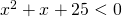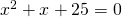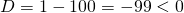# Solution assignment 07 Quadratic inequalities

### Assignment 7

Solve:### Solution

First we solve the corresponding equation:Because the discriminant:the equation has no solutions and thus the parabola has no intersection points with the-axis. Because the parabola 'opens up' it lies above the-axis and thus no values ofsatisfy the inequality.